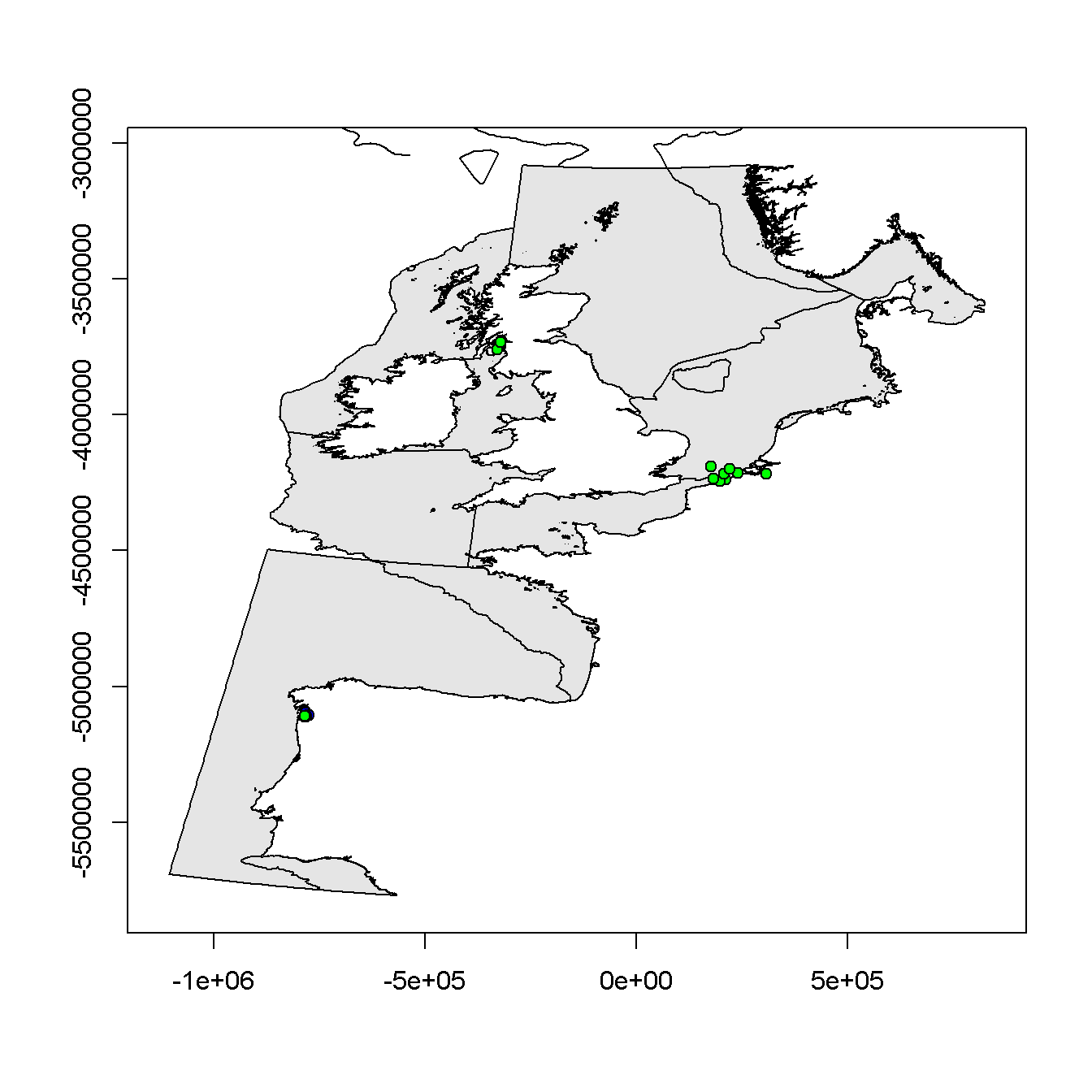## Assessment Criteria for Polybrominated Diphenyl Ethers

This document summarises the discussions at OSPAR MIME 2017 on setting Assessment Criteria for Polybrominated Diphenyl Ethers (PBDEs). In particular, it describes:

• the decision to use Canadian Federal Environmental Quality Guidelines (FEQGs) to assess whether concentrations are ikely to cause harm to marine organisms
• the development of Background Assessment Concentrations (BACs) to assess whether concentrations are ‘close to background’ and the decision to trial BACs for BDE47

EAC equivalents

BAC estimation methodology

BAC estimates

### EAC equivalents

OSPAR Environmental Assessment Criteria (EACs) have not been developed for PBDEs, and are unlikely to be developed in the near future. The few alternatives (that MIME are aware of) with a broadly similar purpose are:

AC substance value tissue (fish) purpose
biota
QS sum BDE28, 47, 99, 100, 153, 154 0.0085 fish muscle human health
QS sum BDE28, 47, 99, 100, 153, 154 44 whole fish secondary poisoning
FEQG total tri BDE (includes BDE28) 120 whole fish fish health
FEQG total tetra BDE (includes BDE47, 66) 88 whole fish fish health
FEQG total tetra BDE (includes BDE47, 66) 44 whole fish mammalian wildlife health
FEQG total penta BDE (includes BDE85, 99, 100) 1 whole fish fish health
FEQG total hexa BDE (includes BD153, 154) 420 whole fish fish health
FEQG total hexa BDE (includes BD153, 154) 4 whole fish mammalian wildlife health
FEQG total hepta BDE (includes BD183) 64 whole fish mammalian wildlife health
FEQG total octa BDE 63 whole fish mammalian wildlife health
FEQG total nona BDE 78 whole fish mammalian wildlife health
FEQG total deca BDE (includes BD209) 9 whole fish mammalian wildlife health
sediment
FEQG total tri BDE (includes BDE28) 44 sediment-dwelling & pelagic health
FEQG total tetra BDE (includes BDE47, 66) 39 sediment-dwelling & pelagic health
FEQG total penta BDE (includes BDE85, 99, 100) 0.4 sediment-dwelling & pelagic health
FEQG total hexa BDE (includes BD153, 154) 440 sediment-dwelling & pelagic health
FEQG total octa BDE 5600 sediment-dwelling & pelagic health
FEQG total deca BDE (includes BD209) 19 sediment-dwelling & pelagic health

The QS are European Commission Environmental Quality Standards. The FEQG are Canadian Federal Environmental Quality Guidelines. Units are $$\mu \text{g kg}^{-1}$$ ww for biota and $$\mu \text{g kg}^{-1}$$ dw for sediment. Sediment concentrations are normalised to 1% organic carbon.

The QS for human health is below the limit of detection of methods often used to measure PBDE concentrations (see later) and was considered by MIME to be too low for meaningful environmental assessments. The QS for secondary poisoning is more environmentally plausible. However, it is based on the sum of six PBDE congeners and was rejected in favour of the FEQGs which relate to specific homologue groups or individual congeners and consequently could provide more stringent assessments for the more toxic homologues. To see this, note that BDE47 usually dominates the PBDE profile in fish and shellfish (and indeed the QS is equal to the FEQG of BDE47 for mammalian wildlife health). Concentrations of the more toxic penta PBDEs that might cause environmental harm could therefore go undetected using the QS because they are masked by the concentration of BDE47.

The FEQGs are based on toxicity tests on a range of organisms, but come with many caveats (see link above).

The FEQGs for biota either relate to fish health (concentrations that should not cause adverse effects on fish) or mammalian wildlife health (concentrations in fish that should not cause adverse effects on mammalian predators). They may not be appropriate for assessing the effect of PBDEs on other groups (such as plants). As such, they might be less protective than an EAC (the concentration that should not cause adverse effects on the most sensitive marine organisms). To counter-balance this, the FEQGs are for whole fish concentrations, which will be lower than the liver concentrations on which MIME assessments are typically based. The FEQGs for sediment relate to the health of sediment-dwelling and pelagic animals and again may not be appropriate for other groups (such as plants).

MIME agreed that the FEQGs could sensibly be applied as EAC-equivalents in the MIME assessment. Doing so (see below and the assessment itself) provided status assessments that were broadly compatible with expectations based on expert knowledge of the monitoring data and of pollution pressures. There are clearly inconsistencies between the FEQGs, the MIME monitoring data, and the underlying principles of an EAC, but these were regarded as small compared to the benefits of having EAC-equivalents that could be used to assess status and to monitor progress towards good environmental health.

To apply the FEQGs, several further decisions were required:

• where an FEQG was available for both fish and mammalian wildlife health, the lower value was chosen
• an FEQG for a homologue was applied to the individual PBDE congeners within that homologue
• biota FEQGs were applied to all fish (typically liver concentrations) and shellfish (soft body concentrations)
• sediment FEQGs were normalised to 2.5% organic carbon (by multiplying by 2.5)
• sediment FEQGs were also applied to non-normalised concentrations (Iberian Sea and Gulf of Cadiz)
• the sediment FEQG for octa BDEs was applied to BD183 (a hepta BDE) since the substance tested in the toxicity tests was a mixture of hepta and octa BDEs

The FEQGs used in the MIME assessment are tabulated below. Units are $$\mu$$g kg$$^{-1}$$ ww for biota and $$\mu$$g kg$$^{-1}$$ dw for sediment. Sediment concentrations are normalised to 2.5% organic carbon. A different suite of PBDEs is typically assessed for biota and sediment, which is reflected in the gaps in the table below.

 biota sediment BDE28 120 110 BDE47 44 97.5 BDE66 97.5 BDE85 1 BDE99 1 1 BD100 1 1 BD153 4 1100 BD154 4 1100 BD183 14000 BD209 47.5

### BAC estimation methodology

BACs are used to test whether concentrations are at background levels for naturally occurring substances, or ‘close to background’ for synthetics. This is done by testing the null hypothesis $${\text{H}_0: \mu \geq \text{BAC}}$$ against the alternative hypothesis $${\text{H}_1: \mu < \text{BAC}}$$, where $$\mu$$ is the mean concentration (in the final monitoring year). The test is precautionary since concentrations are considered to be above background ($${\text{H}_0}$$) unless there is sufficient evidence to show otherwise ($${\text{H}_1}$$).

The BAC is chosen to give a 90% power of rejecting $$\text{H}_0$$ when $$\mu = \text{BC}$$, where BC is the Background Concentration. The BC of natural occurring substances is estimated using data from near-pristine locations (assuming they exist) or, for sediment concentrations, from cores. The BC of synthetic substances is zero, and to construct the BAC it is necessary to replace the BC by a Low Concentration (LC) which is typically taken to be twice the Quasimeme standard error. For the purposes of this section, the BC (or LC) is assumed known.

Power depends on the statistical test used, the number of years of data, and the variability in the data. It is necessary to standardise these to construct a BAC that can applied across the OSPAR area. Specifically, it is assumed that:

• there is a 10-year monitoring programme with the same sampling protocol followed every year (or equivalently and more realistically, a 20-year monitoring programme with sampling every two years)
• the test is a one-tailed t-test at the 5% significance level based on the temporal trend regression model fitted to the monitoring data
• the variability in the data is typical of that found in MIME monitoring data; this is developed later, but for now it is assumed that the variability is characterised by a parameter $$\psi$$

Assume that the concentration measurements each year are summarised by an annual index on the logarithmic scale (e.g. the median log concentration or the mean log concentration). Let $$\mathbf y$$ be the vector (length 10) of annual contaminant indices and assume that

$$\quad \mathbf y = \mathbf f + \mathbf \epsilon$$

where $$\mathbf f$$ represents a smooth systematic trend in contaminant levels over time and $$\mathbf \epsilon$$ represents random variation, assumed to be independent between years and normally distributed with zero mean and standard deviation $$\psi$$. Let $$\mathbf{X}$$ be the design matrix used to estimate the systematic trend $$\mathbf f$$. The choice of $$\mathbf{X}$$ is also discussed later, but it could correspond to a linear trend or a smooth on a fixed number of degrees of freedom. It is assumed that $$\mathbf{X}$$ estimates $$\mathbf f$$ with no bias. Let $$r$$ be the rank of $$\mathbf X$$ and $$\nu = 10 - r$$ be the residual degrees of freedom associated with $$\mathbf X$$. Let

$$\quad \mathbf{H = X(X'X)^{-1}X'}$$

be the hat matrix. The fitted trend is then $$\mathbf {\hat{f} = Hy}$$, which has variance $$\mathbf H \psi^2$$.

A one-tailed t-test at the 5% significance level is used to test $${\text{H}_0}$$ vs $${\text{H}_1}$$. Let

$$\quad T = \frac {\hat {\mathbf f} _{10} \text{ }- \text{log BAC}} {\hat \psi \sqrt {\mathbf H_{10,10}} }$$

where the 10 suffix picks out the fitted value and its variance in the final monitoring year, and where $$\hat {\psi^2}$$ is the usual unbiased estimator of $$\psi^2$$. Then $${\text{H}_0}$$ is rejected in favour of $${\text{H}_1}$$ if $$T < t_{\text{crit}}$$, where $$t_{\text{crit}}$$ is the 0.05 quantile of a central t-distribution on $$\nu$$ degrees of freedom, denoted $$(t ; \nu).$$ That is, the value such that

$$\quad \text{Prob} \big( (t ; \nu) < t_{\text{crit}} \big) = 0.05$$

When $$\mathbf f_{10} = \text{log BAC}$$, $$T$$ has a central t-distribution $$T \sim (t; \nu)$$. More generally, T has a non-central t-distribution $$T \sim (t; \nu; \delta)$$ on $$\nu$$ degrees of freedom and non-centrality parameter

$$\quad \delta = \frac {\mathbf f_{10} - \text{log BAC}} {\psi \sqrt {\mathbf H_{10,10}} }$$

The BAC is chosen to give 90% power when $$\mathbf f_{10} = \text {log BC}$$. Let $$\delta _ \text {crit}$$ be the value that satisfies

$$\quad \text{Prob} \big( (t ; \nu; \delta _ \text{crit} ) < t_{\text{crit}} \big) = 0.90$$

Then the BAC is given by

$$\quad \text {log BAC} = \text {log BC} - \delta _ \text {crit} \psi \sqrt {\mathbf H_{10,10}}$$

or equivalently

$$\quad \text {BAC} = \text {BC} \text {exp} \big( - \delta _ \text {crit} \psi \sqrt {\mathbf H_{10,10}} \big)$$

Two problems remain: choosing the appropriate form of $$\mathbf X$$ and hence $$\mathbf H$$ and estimating a suitable value of $$\psi$$. The methods for doing both have been updated, since the trend assessment methodology has evolved since the last time BACs were constructed (around 2005 for compounds in other determinand groups).

First consider the choice of $$\mathbf X$$. Previously, a loess smoother with a fixed window width was used to estimate $$\mathbf f$$. The consequent values of $$\delta _ \text{crit}$$ and $$\mathbf H$$ resulted in BACs of the form

$$\quad \text {BAC} = \text {BC} \text {exp} (2.31 \psi )$$

Currently, a time series with 10 years of data is assessed by fitting a linear trend and smooths on 2 and 3 degrees of freedom (df) and choosing the optimal model based on AICc. The values of $$\delta _ \text{crit}$$ and $$\mathbf H$$ for these three models give BACs of the form:

• linear trend: $$\text{BAC} = \text{BC}\text{exp}(1.89 \psi)$$
• smooth on 2 df: $$\text{BAC} = \text{BC}\text{exp}(2.51 \psi)$$
• smooth on 3 df: $$\text{BAC} = \text{BC}\text{exp}(2.90 \psi)$$

In practice, most time series reduce to a linear trend. However, the smooth on 2 df gives BACs that are closest to the previous approach and hence were adopted here. That is, BACs were given by

$$\quad \text {BAC} = \text {BC} \text {exp} (2.51 \psi )$$

Now consider the choice of $$\psi$$. Previously, the data were summarised by the annual median log concentration, which were then smoothed over time. This gave an estimate of the residual standard deviation for each time series that implicitly included analytical, between-sample and between-year random variation. Currently, the raw concentration measurements are modelled in a mixed modelling framework. This gives estimates of the between-sample and between-year random variation for each time series, denoted here as $$\sigma_{i,\text {sample}}^2$$ and $$\sigma_{i,\text {year}}^2$$ with the $$i$$ used to index the time series. Analytical variability and the number of samples per year have tended to evolve in most time series (getting lower and fewer respectively) so, to characterise recent performance, the analytical variability $$\sigma_{i,\text {analytical}}^2$$ for time series was based on the median reported uncertainty in the last three monitoring years. Similarly, the number of samples per year $$n_i$$ for time series $$i$$ was taken to be the median number of samples in the last three monitoring years. The residual variance for time series $$i$$ was then estimated to be

$$\quad \psi _i ^2 = \sigma _ {i, \text {year}} ^2 + \frac 1 {n_i} \big (\sigma _ {i, \text {sample}} ^2 + \sigma _ {i, \text {analytical}} ^2 \big )$$

The value of $$\psi$$ typical of MIME monitoring data was then estimated by fitting a robust loess smoother to the $$\text {log} \psi _ i$$ as a function of the fitted log mean concentration in the final monitoring year, with a span of 1 and weighted by the number of years in the time series. The (back-transformed) fitted value of the loess smoother at the BC (LC) is taken to be the typical $$\psi$$ at background (low) concentrations. When the BC (LC) is outside the range of the data, the fitted value at the closest observed mean concentration is used.

One final important caveat. BACs are statistical constructs and there is no guarantee that they are environmentally relevant. They should be rejected if they are higher than the EAC or equivalent (if it exists) and if they are too high based on expert judgement.

### BAC estimates

The layout of the first three sections is as follows:

The first tab shows, by determinand, the estimates of $$\psi$$ from each time series plotted against the estimated mean concentration in the final monitoring year. The points are coloured by contracting party and, for shellfish and fish, by species. The fitted loess smoother (black line) with 95% pointwise confidence limits (dashed lines) is also shown. The three vertical lines are the LC (grey), the BAC (blue) and the FEQG (green).

The second tab gives the estimates of $$\psi$$ typical of MIME monitoring data at concentrations close to the LC, with lower and upper 95% confidence limits, and the corresponding BACs.

The remaining tabs show status plots for each PBDE. Points are coloured

• blue if the mean concentration is significantly (p < 0.05) below the BAC
• green if the mean concentration is significantly (p < 0.05) below the FEQG
• red if the mean concentration is not significantly below the FEQG
• orange if there is no FEQG (and above background)

Details specific to sediment, shellfish and fish are given in the relevant section.

The conclusions are in the concluding section.

#### Sediment

The Quasimeme constant error is 0.05 $$\mu$$g/kg dw for all determinands, so the LC is taken to be 0.1 $$\mu$$g/kg dw.

Mean concentrations in the final monitoring year are normalised to 2.5% organic carbon for Belgium, the Netherlands, Sweden and the United Kingdom but are not normalised for Spain. There are systematic differences in the individual time series estimates of $$\psi$$ between contracting parties, which could be due to differences in the number of samples taken, analytical and reporting quality control, or natural variability in concentrations due to choice of monitoring stations or other environmental drivers.

There were too few data to estimate a BAC for BDE85.

The BACs are below the FEQGs in all cases. However, the BACs for BDE99 and BD100 are relatively close to the corresponding FEQGs. (Note the FEQG for BD183 is not plotted because it is off the scale of the plot.)

The BACs are expressed as $$\mu$$g/kg dw normalised to 2.5% organic carbon for normalised sediments and $$\mu$$g/kg dw for non-normalised sediments

##### Variability by country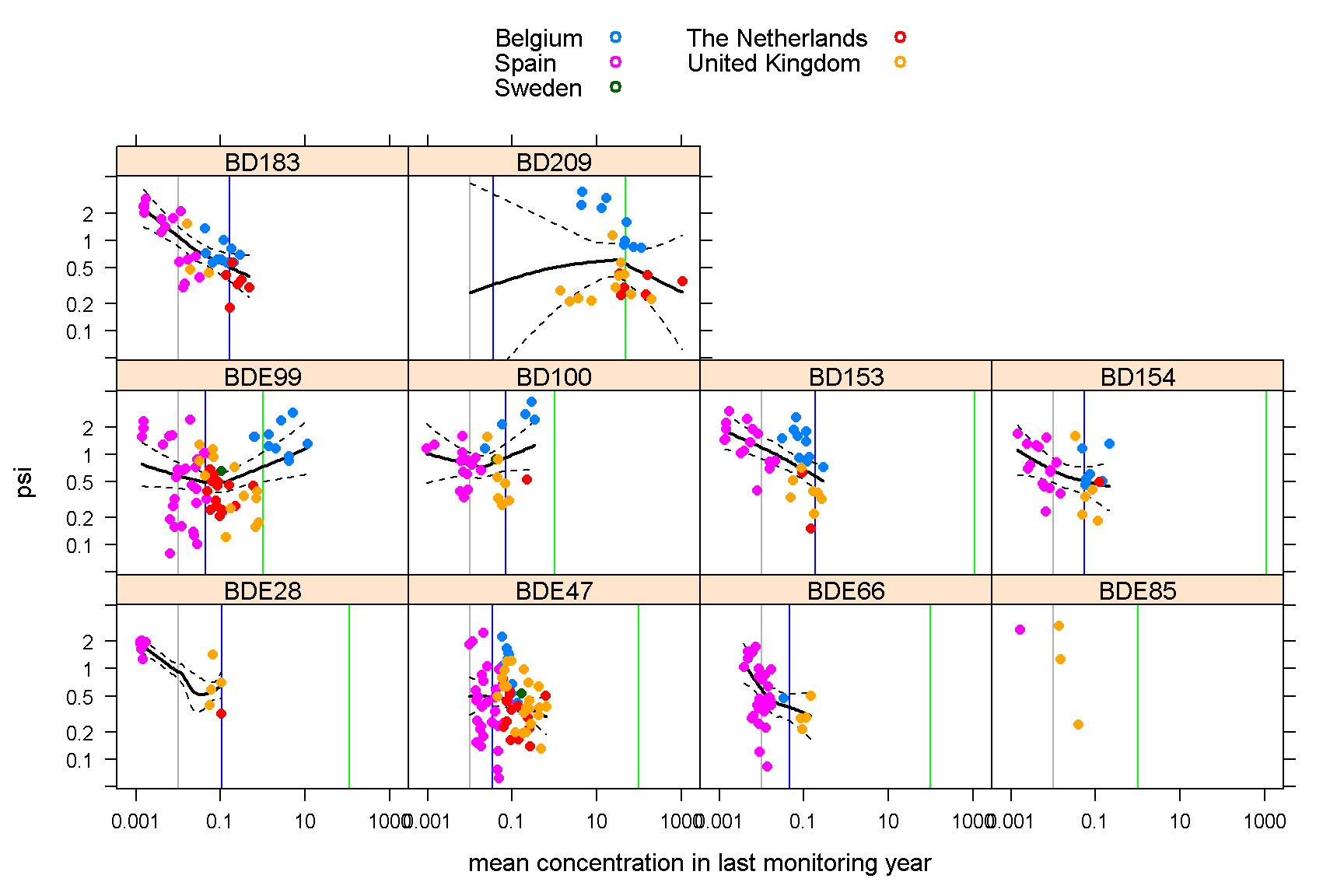##### BACs
LC psi lower upper BAC FEQG
BDE28 0.01 0.92 0.77 1.10 0.10 110.0
BDE47 0.01 0.50 0.31 0.80 0.04 97.5
BDE66 0.01 0.59 0.46 0.77 0.04 97.5
BDE85 0.01 1.0
BDE99 0.01 0.58 0.42 0.81 0.04 1.0
BD100 0.01 0.77 0.57 1.05 0.07 1.0
BD153 0.01 1.19 0.91 1.57 0.20 1100.0
BD154 0.01 0.67 0.49 0.91 0.05 1100.0
BD183 0.01 1.10 0.86 1.43 0.16 14000.0
BD209 0.01 0.52 0.19 1.43 0.04 47.5

##### BDE28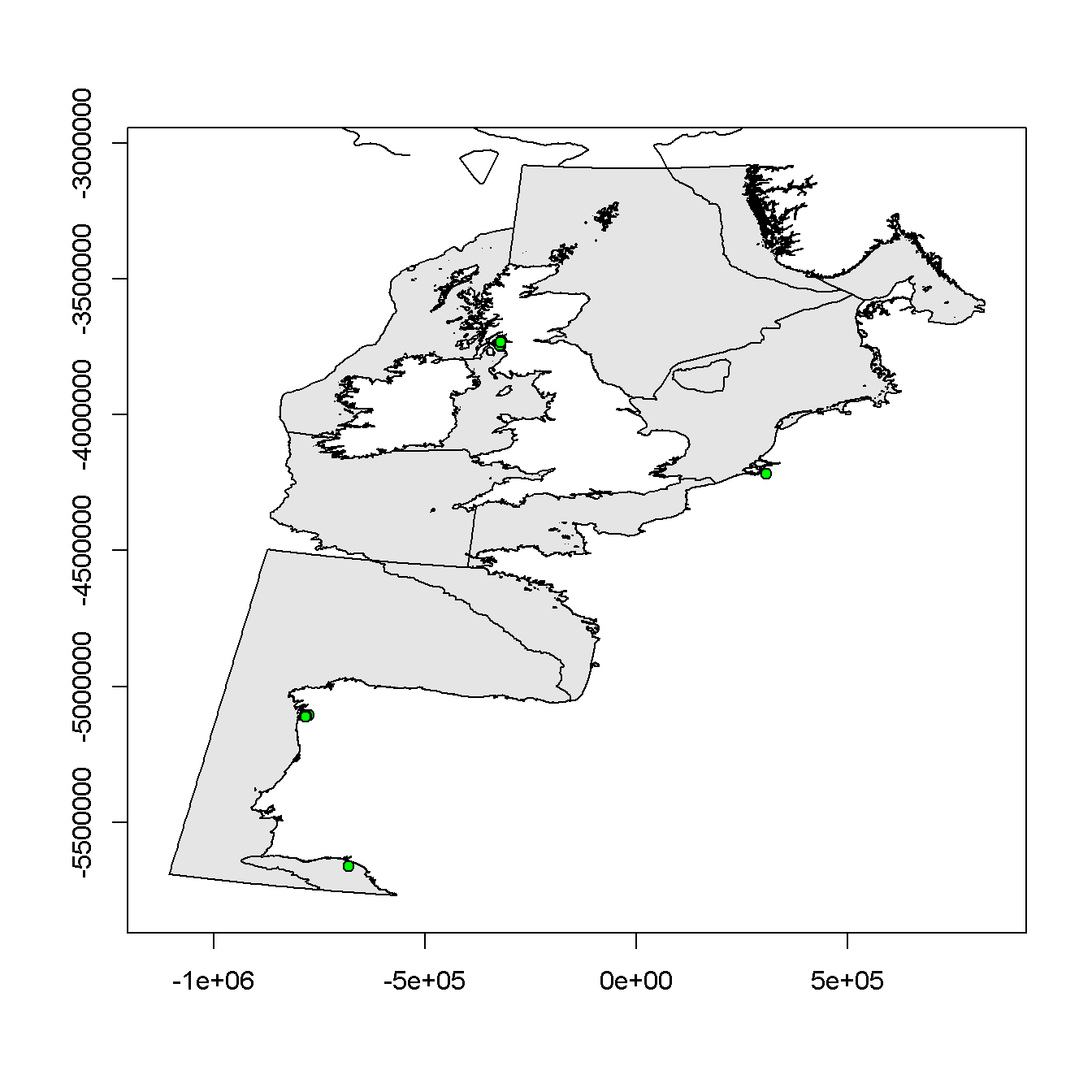##### BDE47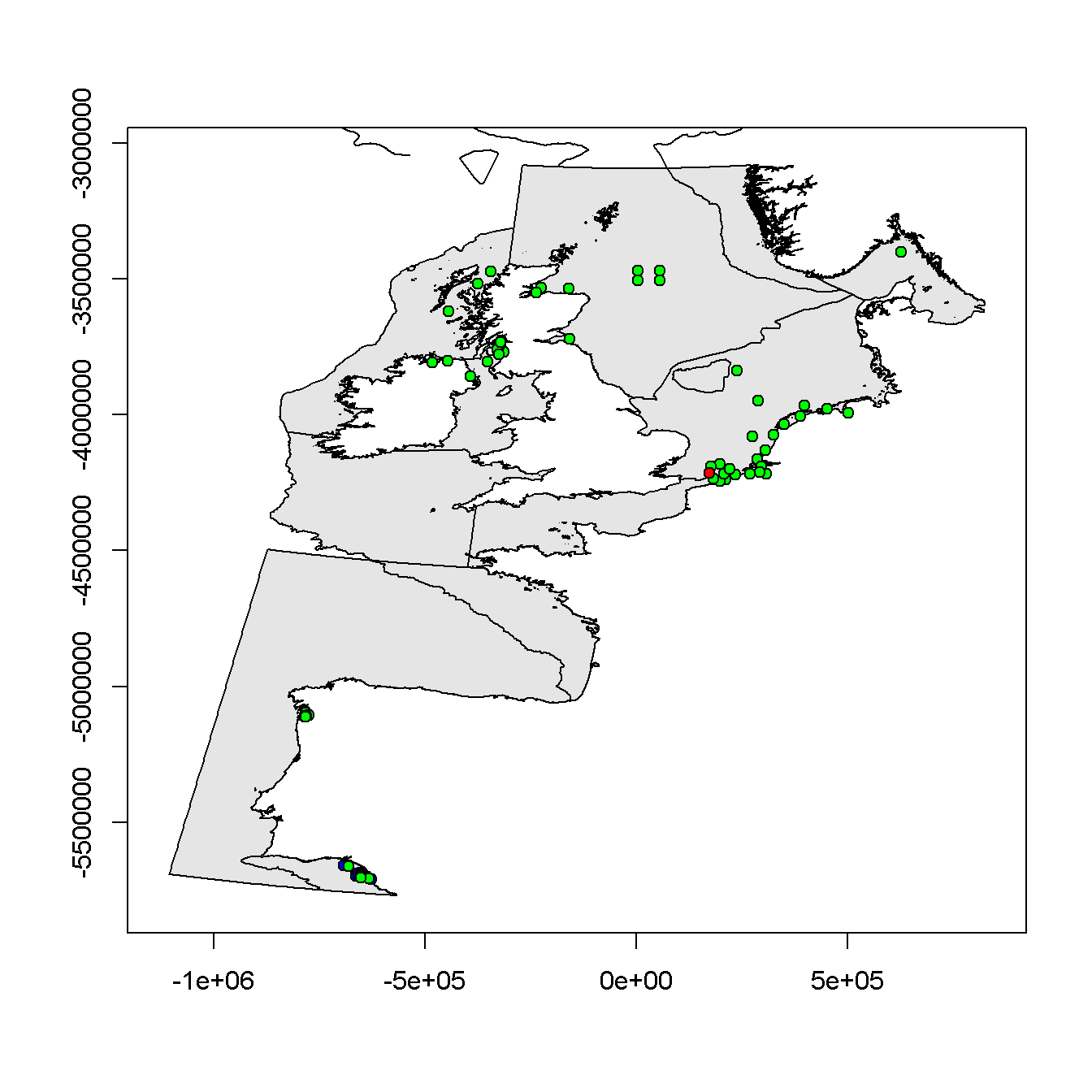##### BDE66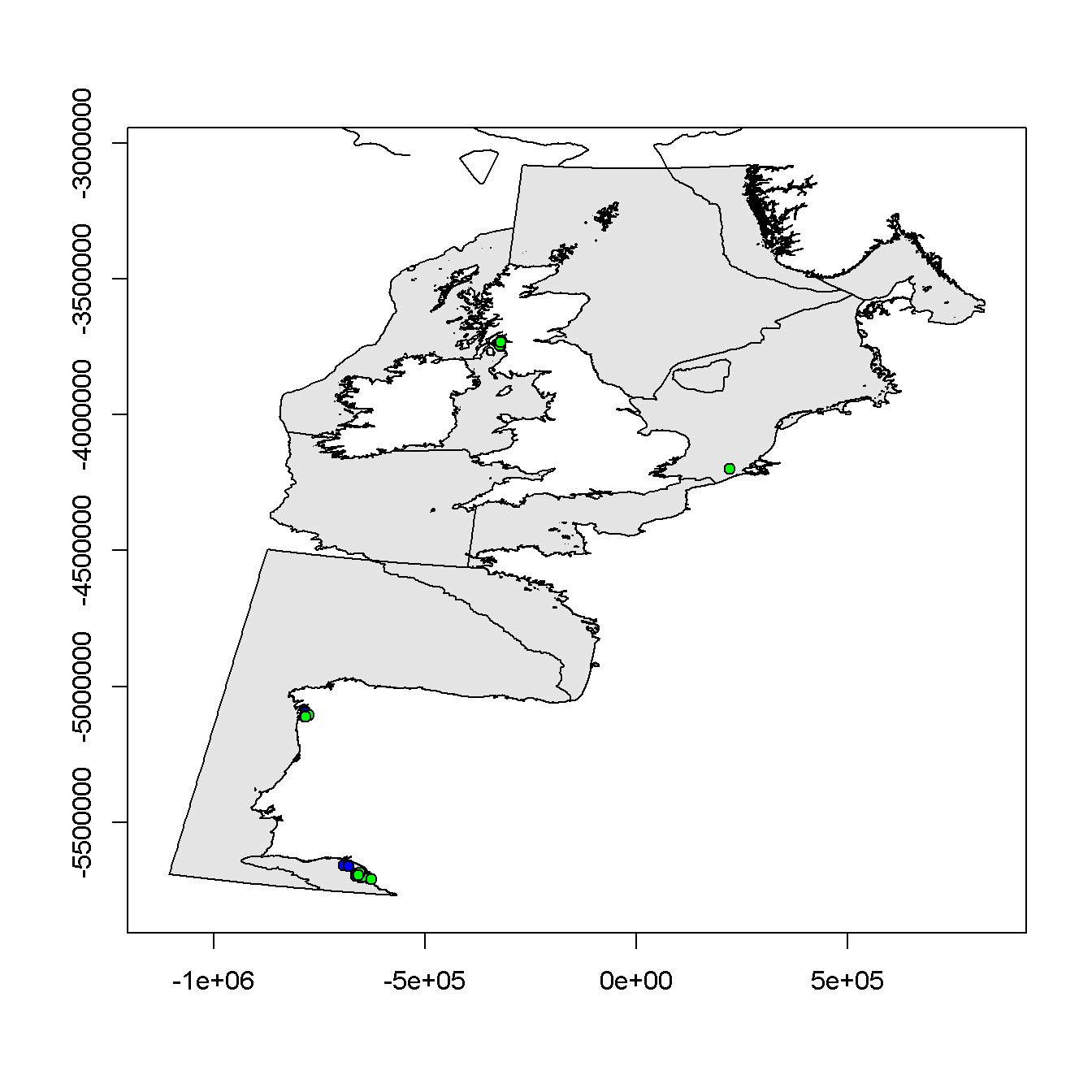##### BDE99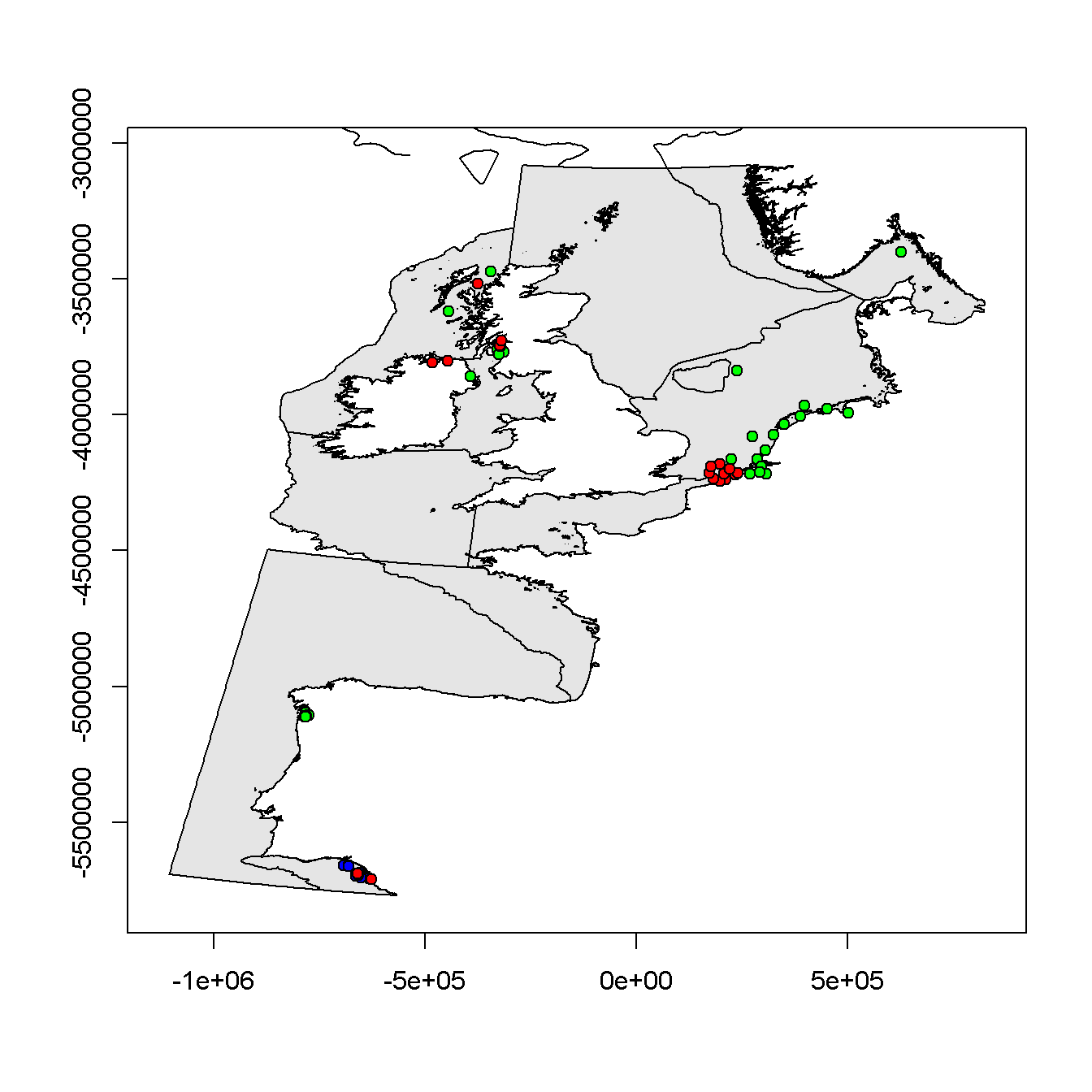##### BD100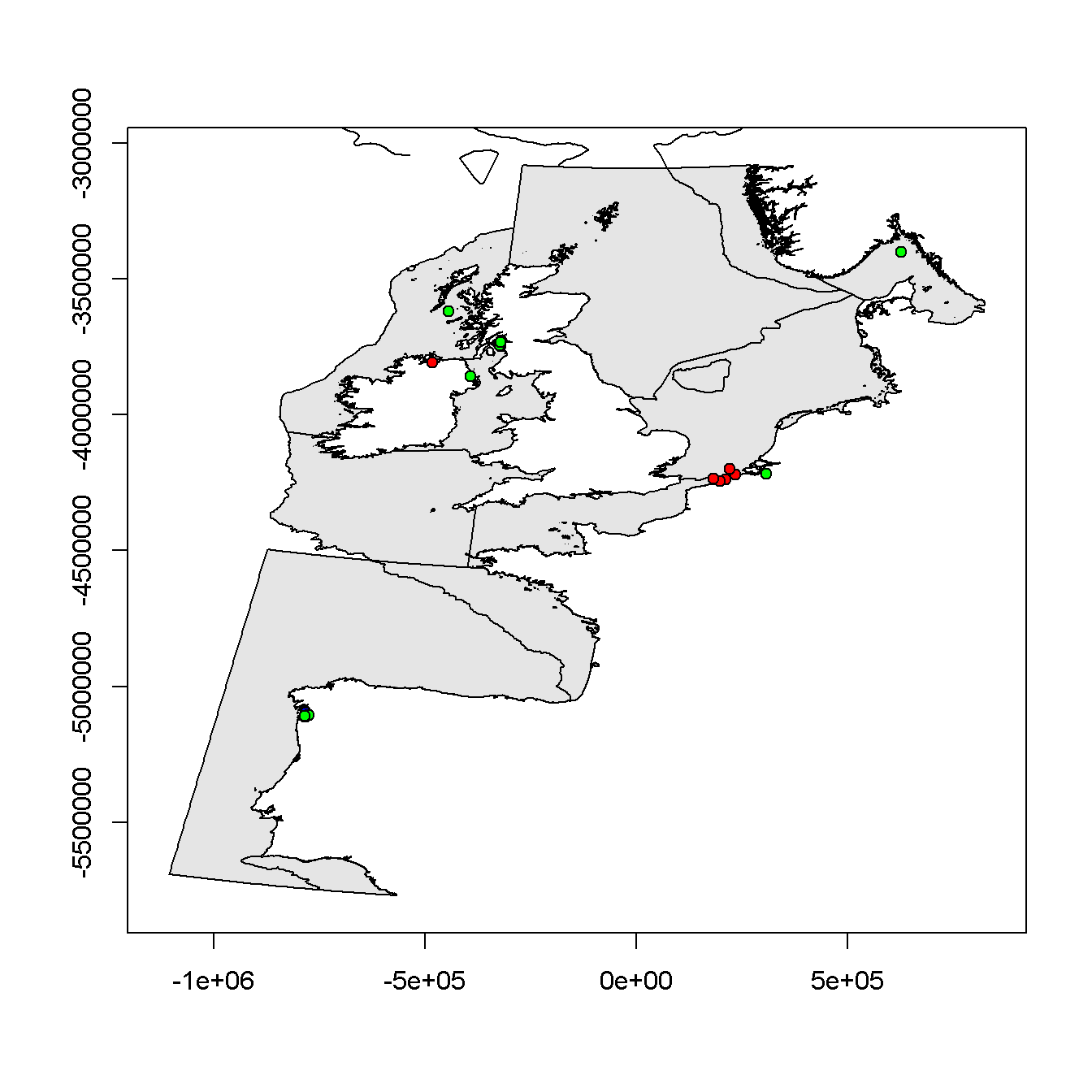##### BD153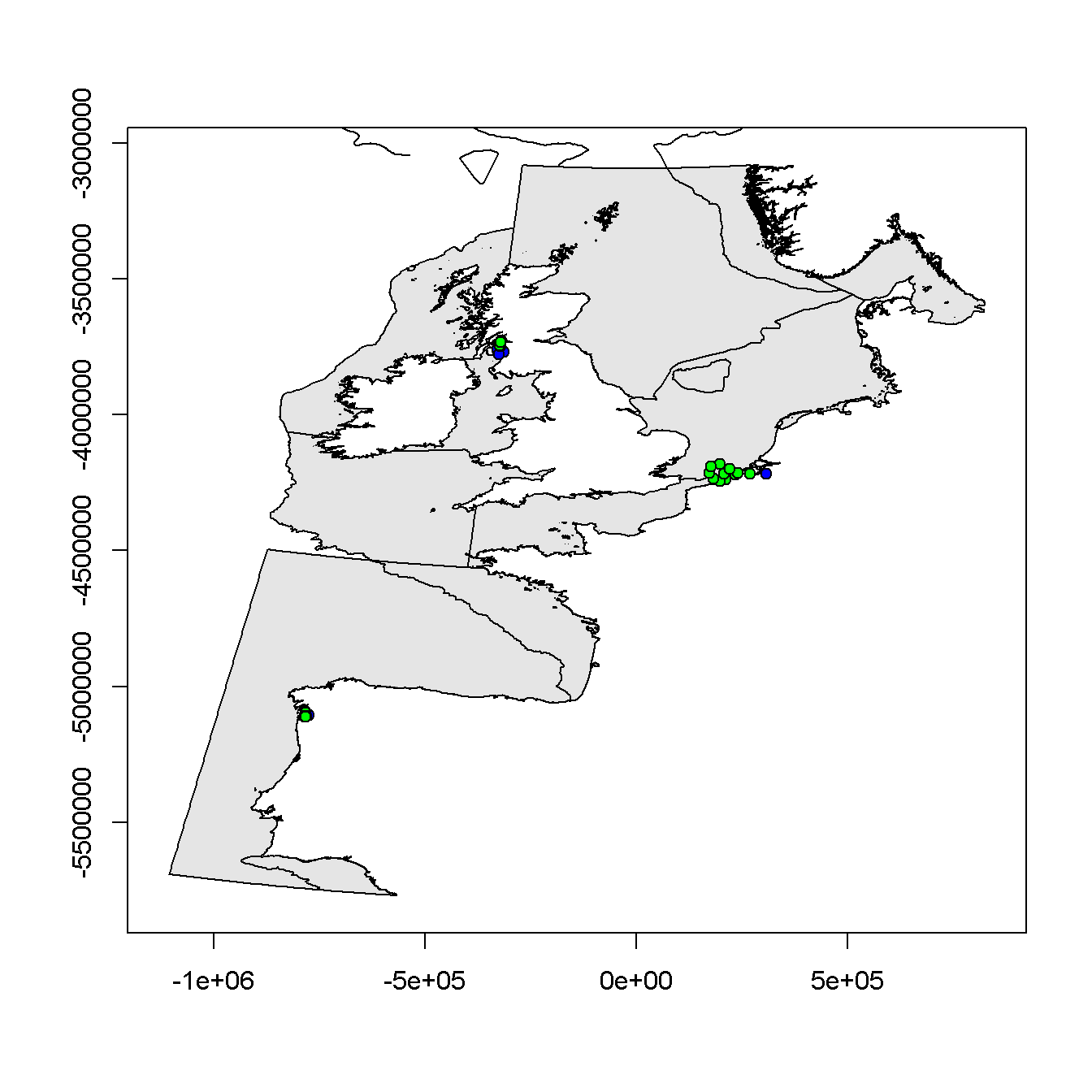##### BD154